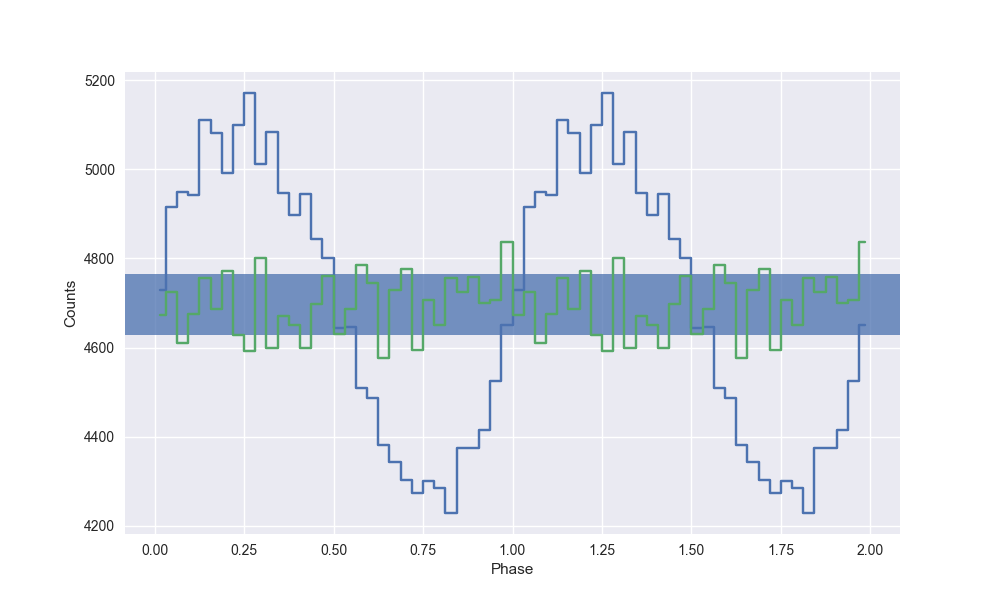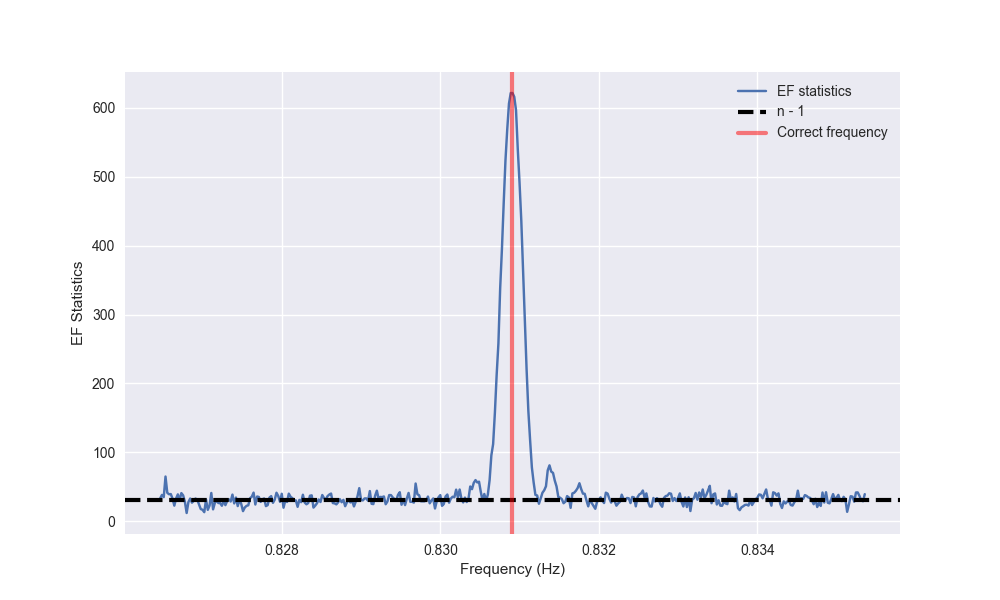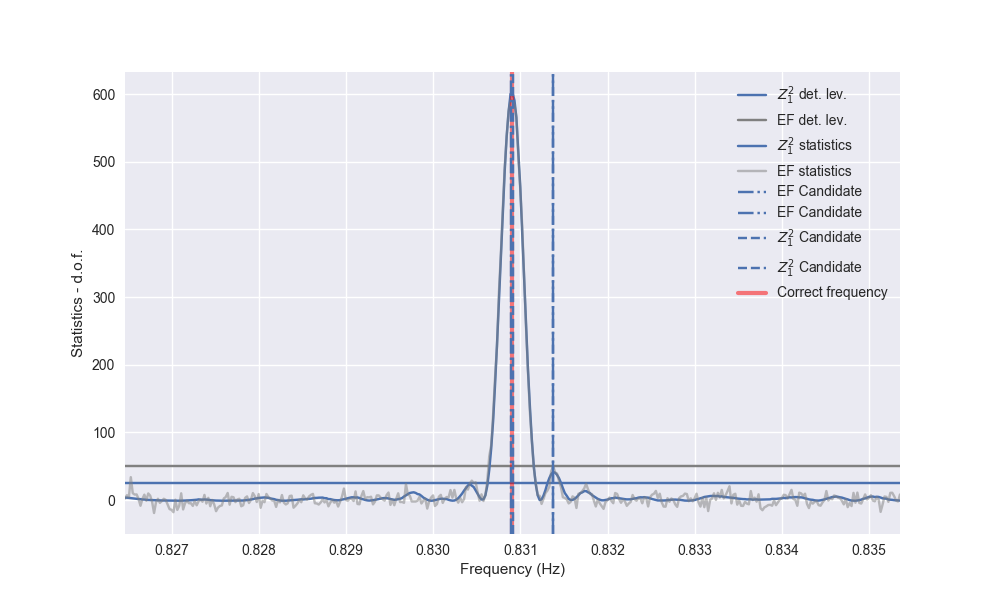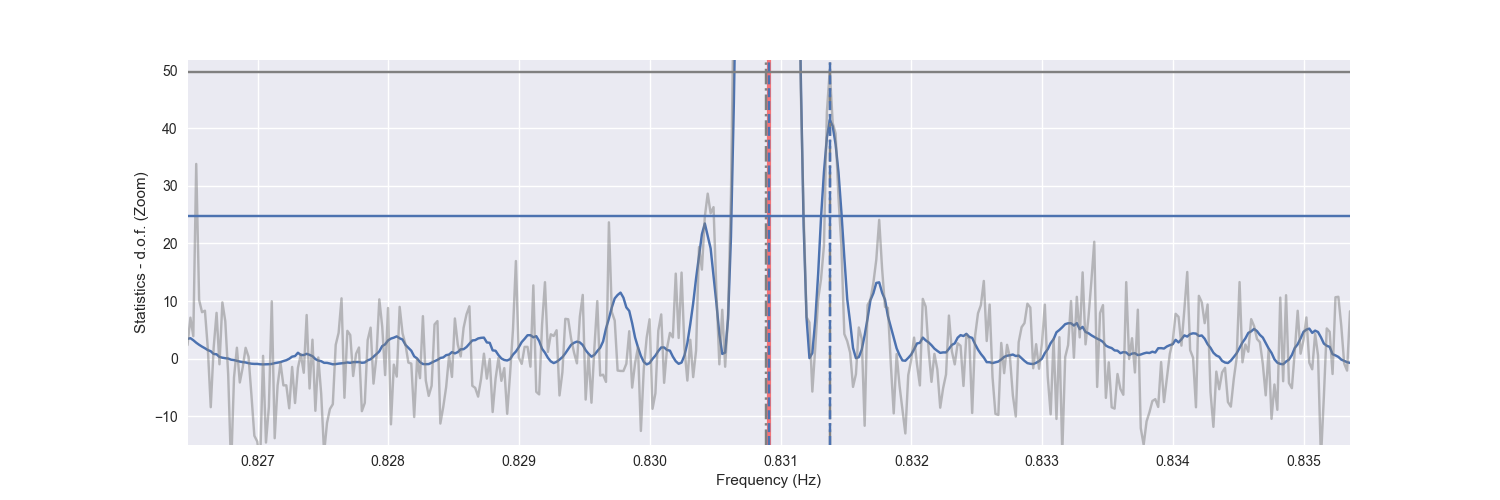:

%load_ext autoreload
%matplotlib notebook

import numpy as np
from stingray.pulse.search import epoch_folding_search, z_n_search
import matplotlib.pyplot as plt
import seaborn as sb
import matplotlib as mpl
mpl.rcParams['figure.figsize'] = (10, 6)


# Simulate a dataset¶

Let us simulate a pulsar: we create a sinusoidal light curve and use Stingray’s event simulator (in Eventlist.simulate_times) to simulate an event list with that light curve.

:

def sinusoid(times, frequency, baseline, amplitude, phase):
return baseline + amplitude * np.sin(2 * np.pi * (frequency * times + phase))

:

from stingray import Lightcurve

period = 1.203501
mean_countrate = 50
pulsed_fraction = 0.2
bin_time = 0.01
obs_length = 3000

t = np.arange(0, obs_length, bin_time)

# The continuous light curve
counts = sinusoid(t, 1 / period, mean_countrate,
0.5 * mean_countrate * pulsed_fraction, 0) * bin_time
lc = Lightcurve(t, counts, gti=[[-bin_time / 2, obs_length + bin_time / 2]],
dt=bin_time)

:

from stingray.events import EventList

# use the light curve above to simulate an event list for this pulsar.
events = EventList()
events.simulate_times(lc)


# Pulsation search with epoch folding.¶

Let us assume we have already an estimate of the pulse period, for example because we found a candidate in the power density spectrum with a period of ~1.2. We search around that period with the epoch folding.

Epoch folding consists of cutting the light curve at every pulse period and summing up all the intervals obtained in this way. We get an average pulse profile. In this example,

:

from stingray.pulse.pulsar import fold_events
from stingray.pulse.search import plot_profile
nbin = 32

ph, profile, profile_err = fold_events(events.time, 1/period, nbin=nbin)

_ = plot_profile(ph, profile)where the pulse was plotted twice for visual clarity. If the candidate pulse frequency was even slightly incorrect, we would have obtained a much shallower pulse profile, or no pulse profile at all. E.g.

:

ph, profile, profile_err = fold_events(events.time, 1/1.1, nbin=nbin)

_ = plot_profile(ph, profile)


Therefore, typically we try a number of frequencies around the candidate we found with the power spectrum or other means, and search for the frequency that gives the “best” pulsed profile. How do we evaluate this best frequency? We use the chi squared statistics.

We use a flat pulsed profile (no pulsation) as model, and we calculate the chi square of the actual pulsed profile with respect to this flat model:

$S = \sum_i\frac{(P_i - \overline{P})^2}{\sigma^2}$

If there is no pulsation, the chi squared will assume a random value distributed around the number of degrees of freedom $$n - 1$$ (where $$n$$ is the number of bins in the profile) with a well defined statistical distribution ($$\chi^2_{n - 1}$$). If there is pulsation, the value will be much larger. Stingray has a function that does this: stingray.pulse.search.epoch_folding_search.

For the frequency resolution of the periodogram, one usually chooses at least the same frequency resolution of the FFT, i. e., $$df_{\rm min}=1/(t_1 - t_0)$$. In most cases, a certain degree of oversampling is used.

:

# We will search for pulsations over a range of frequencies around the known pulsation period.
df_min = 1/obs_length
oversampling=15
df = df_min / oversampling
frequencies = np.arange(1/period - 200 * df, 1/period + 200 * df, df)

freq, efstat = epoch_folding_search(events.time, frequencies, nbin=nbin)

# ---- PLOTTING --------
plt.figure()
plt.plot(freq, efstat, label='EF statistics')
plt.axhline(nbin - 1, ls='--', lw=3, color='k', label='n - 1')
plt.axvline(1/period, lw=3, alpha=0.5, color='r', label='Correct frequency')
plt.xlabel('Frequency (Hz)')
plt.ylabel('EF Statistics')
_ = plt.legend()A peak is definitely there. Far from the peak, the periodogram follows approximately a :math:chi^2 distribution with :math:n - 1 degrees of freedom, where $$n$$ is the number of bins in the pulse profile used to calculate the statistics. In fact, its mean is $$n-1$$ as shown in the figure.

But close to the correct frequency, as described in Leahy et al. 1983, 1987 the peak in the epoch folding periodogram has the shape of a sinc squared function (whose secondary lobes are in this case barely visible above noise).

# Thresholding¶

When can a peak in the EF or $$Z_n^2$$ periodogram be considered a pulsation?

Since both the EF and $$Z_n^2$$ of noise follow precise statistical distributions ($$\chi^2_{\rm nbin}$$ in one case, $$\chi^2_n$$ in the other), we can use the inverse survival functions of these statistical distributions to find the peaks that are not expected by noise.

In Stingray, the thresholds are defined in stingray.pulse.pulsar.fold_detection_level and stingray.pulse.pulsar.z2_n_detection_level respectively.

The ntrial parameter should be set to an estimate of the statistically independent frequencies in the periodogram. A good estimate can be

$N_{\rm trial} \sim (f_{\rm max} - f_{\rm min}) / df_{\rm min} =(f_{\rm max} - f_{\rm min}) (t_1 - t_0)$

, where $$f_{\rm min}$$ and $$f_{\rm max}$$ are the maximum and minimum frequencies of the periodogram, $$df_{\rm min}$$ was defined above and $$t_0$$ ans $$t_1$$ the start and end of the observation.

Moreover, the stingray.pulse.search.search_best_peaks helps finding the best value for nearby candidates.

:

from stingray.pulse.search import search_best_peaks
from stingray.pulse.pulsar import fold_detection_level, z2_n_detection_level

ntrial = (frequencies[-1] - frequencies) / df_min
z_detlev = z2_n_detection_level(n=1, epsilon=0.001, ntrial=len(freq))
ef_detlev = fold_detection_level(nbin, epsilon=0.001, ntrial=len(freq))

cand_freqs_ef, cand_stat_ef = search_best_peaks(freq, efstat, ef_detlev)
cand_freqs_z, cand_stat_z = search_best_peaks(freq, zstat, z_detlev)

# ---- PLOTTING --------
plt.figure()
plt.axhline(z_detlev - nharm, label='$Z^2_1$ det. lev.')
plt.axhline(ef_detlev - nbin + 1, label='EF det. lev.', color='gray')

plt.plot(freq, (zstat - nharm), label='$Z^2_1$ statistics')
plt.plot(freq, efstat - nbin + 1, color='gray', label='EF statistics', alpha=0.5)

for c in cand_freqs_ef:
plt.axvline(c, ls='-.', label='EF Candidate', zorder=10)
for c in cand_freqs_z:
plt.axvline(c, ls='--', label='$Z^2_1$ Candidate', zorder=10)

plt.axvline(1/period, color='r', lw=3, alpha=0.5, label='Correct frequency')
plt.xlim([frequencies, frequencies[-1]])
plt.xlabel('Frequency (Hz)')
plt.ylabel('Statistics - d.o.f.')
plt.legend()

plt.figure(figsize=(15, 5))
plt.plot(freq, (zstat - nharm), label='$Z_2$ statistics')
plt.plot(freq, efstat - nbin + 1, color='gray', label='EF statistics', alpha=0.5)

plt.axvline(1/period, color='r', lw=3, alpha=0.5, label='Correct frequency')
plt.axhline(z_detlev - nharm, label='$Z^2_1$ det. lev.', zorder=10)
plt.axhline(ef_detlev - nbin + 1, label='EF det. lev.', color='gray', zorder=10)

for c in cand_freqs_ef:
plt.axvline(c, ls='-.', label='EF Candidate', color='gray', zorder=10)
for c in cand_freqs_z:
plt.axvline(c, ls='--', label='$Z^2_1$ Candidate', zorder=10)

plt.xlabel('Frequency (Hz)')
plt.ylabel('Statistics - d.o.f. (Zoom)')

plt.ylim([-15, ef_detlev - nbin + 3])
_ = plt.xlim([frequencies, frequencies[-1]])Note that the side lobes of the sinc squared-like shape are producing spurious candidates here. For now, we do not have a method to eliminate these fairly obvious patterns, but it will be implemented in future releases

# Fit peak with Sinc-squared and Gaussian functions¶

As we saw earlier, if the pulse frequency is stable during the observation, the peak shape is a Sinc squared function. Therefore we fit it to the peak with the function stingray.pulse.modeling.fit_sinc.
We have two possibilities:
• if obs_length is the length of the observation. If it is defined, it fixes width to $$1/(\pi*obs length)$$, as expected from epoch folding periodograms. The other two free parameters are amplitude and mean.

• if it is not defined, the width parameter can be used.

On the other hand, if the pulse frequency varies slightly, the peak oscillate and the integrated profile is a bell-shaped function. We can fit it with a Gaussian function (stingray.pulse.modeling.fit_gaussian) with the standard parameters: amplitude, mean, stddev.

We also provide the user with the constrains fixed, tied, bounds, in order to fix, link and/or constrain parameters.

:

from stingray.pulse.modeling import fit_sinc

fs=fit_sinc(freq, efstat-(nbin-1),amp=max(efstat-(nbin-1)), mean=cand_freqs_ef,
obs_length=obs_length)

:

# ---- PLOTTING --------
plt.figure()
plt.plot(freq, efstat-(nbin-1), label='EF statistics')
plt.plot(freq, fs(freq), label='Best fit')
plt.axvline(1/period, lw=3, alpha=0.5, color='r', label='Correct frequency')
plt.axvline(fs.mean, label='Fit frequency')

plt.xlabel('Frequency (Hz)')
plt.ylabel('EF Statistics')
plt.legend()

plt.figure(figsize=(15, 5))
plt.plot(freq, efstat-(nbin-1)-fs(freq))
plt.xlabel('Frequency (Hz)')
_ = plt.ylabel('Residuals')